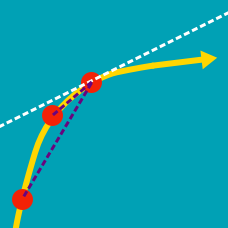Calculus

# Derivatives of Exponential Functions

What is the derivative of the function $y=4e^x?$

What is the derivative of the function $y=e^{3x}?$

What is the derivative of the function $y=3^{x}?$

What is the derivative of the function $y=6^{2x}?$

What is the derivative of the function $y=x^{3}+9e^x?$

×